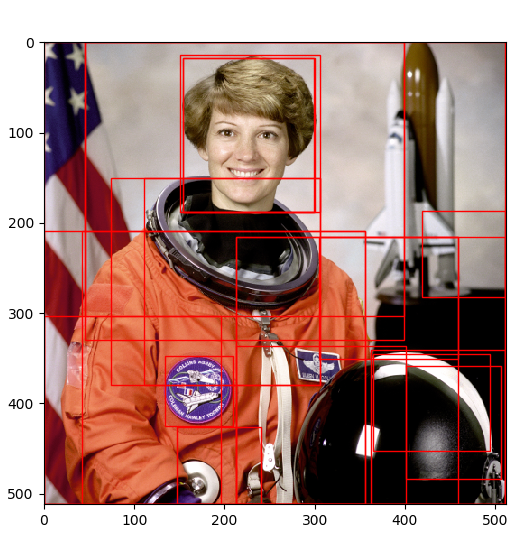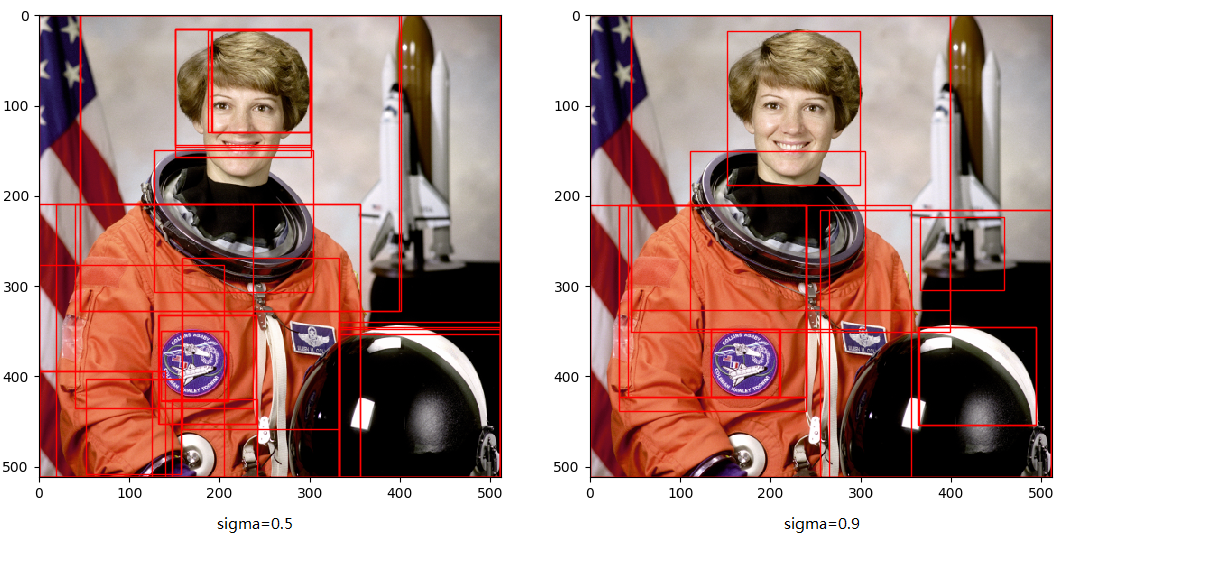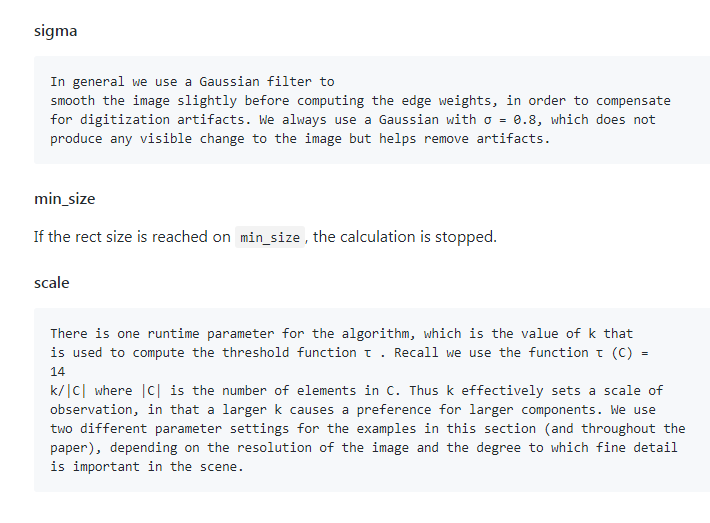# 一、selective search python实现

# -*- coding: utf-8 -*-
from __future__ import (
division,
print_function,
)

import skimage.data
import matplotlib.pyplot as plt
import matplotlib.patches as mpatches
import selectivesearch

def main():

img = skimage.data.astronaut()

# perform selective search
img_lbl, regions = selectivesearch.selective_search(
img, scale=500, sigma=0.8, min_size=10)

candidates = set()
for r in regions:
# excluding same rectangle (with different segments)
if r['rect'] in candidates:
continue
# excluding regions smaller than 2000 pixels
if r['size'] < 2000:
continue
# distorted rects
x, y, w, h = r['rect']   #x,y,w,h 分别代表矩形左下角坐标、长、宽
if w / h > 1.2 or h / w > 1.2:
continue

# draw rectangles on the original image
fig, ax = plt.subplots(ncols=1, nrows=1, figsize=(6, 6))
ax.imshow(img)
for x, y, w, h in candidates:
print(x, y, w, h)
rect = mpatches.Rectangle(
(x, y), w, h, fill=False, edgecolor='red', linewidth=1)

plt.show()

if __name__ == "__main__":
main()


# -*- coding: utf-8 -*-
from __future__ import division

import skimage.io
import skimage.feature
import skimage.color
import skimage.transform
import skimage.util
import skimage.segmentation
import numpy

# "Selective Search for Object Recognition" by J.R.R. Uijlings et al.
#
#  - Modified version with LBP extractor for texture vectorization

def _generate_segments(im_orig, scale, sigma, min_size):
"""
segment smallest regions by the algorithm of Felzenswalb and
Huttenlocher
"""

# open the Image
skimage.util.img_as_float(im_orig), scale=scale, sigma=sigma,
min_size=min_size)

# merge mask channel to the image as a 4th channel
im_orig = numpy.append(
im_orig, numpy.zeros(im_orig.shape[:2])[:, :, numpy.newaxis], axis=2)

return im_orig

def _sim_colour(r1, r2):
"""
calculate the sum of histogram intersection of colour
"""
return sum([min(a, b) for a, b in zip(r1["hist_c"], r2["hist_c"])])

def _sim_texture(r1, r2):
"""
calculate the sum of histogram intersection of texture
"""
return sum([min(a, b) for a, b in zip(r1["hist_t"], r2["hist_t"])])

def _sim_size(r1, r2, imsize):
"""
calculate the size similarity over the image
"""
return 1.0 - (r1["size"] + r2["size"]) / imsize

def _sim_fill(r1, r2, imsize):
"""
calculate the fill similarity over the image
"""
bbsize = (
(max(r1["max_x"], r2["max_x"]) - min(r1["min_x"], r2["min_x"]))
* (max(r1["max_y"], r2["max_y"]) - min(r1["min_y"], r2["min_y"]))
)
return 1.0 - (bbsize - r1["size"] - r2["size"]) / imsize

def _calc_sim(r1, r2, imsize):
return (_sim_colour(r1, r2) + _sim_texture(r1, r2)
+ _sim_size(r1, r2, imsize) + _sim_fill(r1, r2, imsize))

def _calc_colour_hist(img):
"""
calculate colour histogram for each region

the size of output histogram will be BINS * COLOUR_CHANNELS(3)

number of bins is 25 as same as [uijlings_ijcv2013_draft.pdf]

extract HSV
"""

BINS = 25
hist = numpy.array([])

for colour_channel in (0, 1, 2):

# extracting one colour channel
c = img[:, colour_channel]

# calculate histogram for each colour and join to the result
hist = numpy.concatenate(
[hist] + [numpy.histogram(c, BINS, (0.0, 255.0))])

# L1 normalize
hist = hist / len(img)

return hist

"""
calculate texture gradient for entire image

The original SelectiveSearch algorithm proposed Gaussian derivative
for 8 orientations, but we use LBP instead.

output will be [height(*)][width(*)]
"""
ret = numpy.zeros((img.shape, img.shape, img.shape))

for colour_channel in (0, 1, 2):
ret[:, :, colour_channel] = skimage.feature.local_binary_pattern(
img[:, :, colour_channel], 8, 1.0)

return ret

def _calc_texture_hist(img):
"""
calculate texture histogram for each region

calculate the histogram of gradient for each colours
the size of output histogram will be
BINS * ORIENTATIONS * COLOUR_CHANNELS(3)
"""
BINS = 10

hist = numpy.array([])

for colour_channel in (0, 1, 2):

# mask by the colour channel
fd = img[:, colour_channel]

# calculate histogram for each orientation and concatenate them all
# and join to the result
hist = numpy.concatenate(
[hist] + [numpy.histogram(fd, BINS, (0.0, 1.0))])

# L1 Normalize
hist = hist / len(img)

return hist

def _extract_regions(img):

R = {}

# get hsv image
hsv = skimage.color.rgb2hsv(img[:, :, :3])

# pass 1: count pixel positions
for y, i in enumerate(img):

for x, (r, g, b, l) in enumerate(i):

# initialize a new region
if l not in R:
R[l] = {
"min_x": 0xffff, "min_y": 0xffff,
"max_x": 0, "max_y": 0, "labels": [l]}

# bounding box
if R[l]["min_x"] > x:
R[l]["min_x"] = x
if R[l]["min_y"] > y:
R[l]["min_y"] = y
if R[l]["max_x"] < x:
R[l]["max_x"] = x
if R[l]["max_y"] < y:
R[l]["max_y"] = y

# pass 2: calculate texture gradient

# pass 3: calculate colour histogram of each region
for k, v in list(R.items()):

# colour histogram
masked_pixels = hsv[:, :, :][img[:, :, 3] == k]

# texture histogram
R[k]["hist_t"] = _calc_texture_hist(tex_grad[:, :][img[:, :, 3] == k])

return R

def _extract_neighbours(regions):

def intersect(a, b):
if (a["min_x"] < b["min_x"] < a["max_x"]
and a["min_y"] < b["min_y"] < a["max_y"]) or (
a["min_x"] < b["max_x"] < a["max_x"]
and a["min_y"] < b["max_y"] < a["max_y"]) or (
a["min_x"] < b["min_x"] < a["max_x"]
and a["min_y"] < b["max_y"] < a["max_y"]) or (
a["min_x"] < b["max_x"] < a["max_x"]
and a["min_y"] < b["min_y"] < a["max_y"]):
return True
return False

R = list(regions.items())
neighbours = []
for cur, a in enumerate(R[:-1]):
for b in R[cur + 1:]:
if intersect(a, b):
neighbours.append((a, b))

return neighbours

def _merge_regions(r1, r2):
new_size = r1["size"] + r2["size"]
rt = {
"min_x": min(r1["min_x"], r2["min_x"]),
"min_y": min(r1["min_y"], r2["min_y"]),
"max_x": max(r1["max_x"], r2["max_x"]),
"max_y": max(r1["max_y"], r2["max_y"]),
"size": new_size,
"hist_c": (
r1["hist_c"] * r1["size"] + r2["hist_c"] * r2["size"]) / new_size,
"hist_t": (
r1["hist_t"] * r1["size"] + r2["hist_t"] * r2["size"]) / new_size,
"labels": r1["labels"] + r2["labels"]
}
return rt

def selective_search(
im_orig, scale=1.0, sigma=0.8, min_size=50):
'''Selective Search

Parameters
----------
im_orig : ndarray
Input image
scale : int
Free parameter. Higher means larger clusters in felzenszwalb segmentation.
sigma : float
Width of Gaussian kernel for felzenszwalb segmentation.
min_size : int
Minimum component size for felzenszwalb segmentation.
Returns
-------
img : ndarray
image with region label
region label is stored in the 4th value of each pixel [r,g,b,(region)]
regions : array of dict
[
{
'rect': (left, top, width, height),
'labels': [...],
'size': component_size
},
...
]
'''
assert im_orig.shape == 3, "3ch image is expected"

# load image and get smallest regions
# region label is stored in the 4th value of each pixel [r,g,b,(region)]
img = _generate_segments(im_orig, scale, sigma, min_size)

if img is None:
return None, {}

imsize = img.shape * img.shape
R = _extract_regions(img)

# extract neighbouring information
neighbours = _extract_neighbours(R)

# calculate initial similarities
S = {}
for (ai, ar), (bi, br) in neighbours:
S[(ai, bi)] = _calc_sim(ar, br, imsize)

# hierarchal search
while S != {}:

# get highest similarity
i, j = sorted(S.items(), key=lambda i: i)[-1]

# merge corresponding regions
t = max(R.keys()) + 1.0
R[t] = _merge_regions(R[i], R[j])

# mark similarities for regions to be removed
key_to_delete = []
for k, v in list(S.items()):
if (i in k) or (j in k):
key_to_delete.append(k)

# remove old similarities of related regions
for k in key_to_delete:
del S[k]

# calculate similarity set with the new region
for k in [a for a in key_to_delete if a != (i, j)]:
n = k if k in (i, j) else k
S[(t, n)] = _calc_sim(R[t], R[n], imsize)

regions = []
for k, r in list(R.items()):
regions.append({
'rect': (
r['min_x'], r['min_y'],
r['max_x'] - r['min_x'], r['max_y'] - r['min_y']),
'size': r['size'],
'labels': r['labels']
})

return img, regions04-23567
07-2437008-161108
08-046948
11-105万+
06-275958
06-275099
04-241万+
06-037538